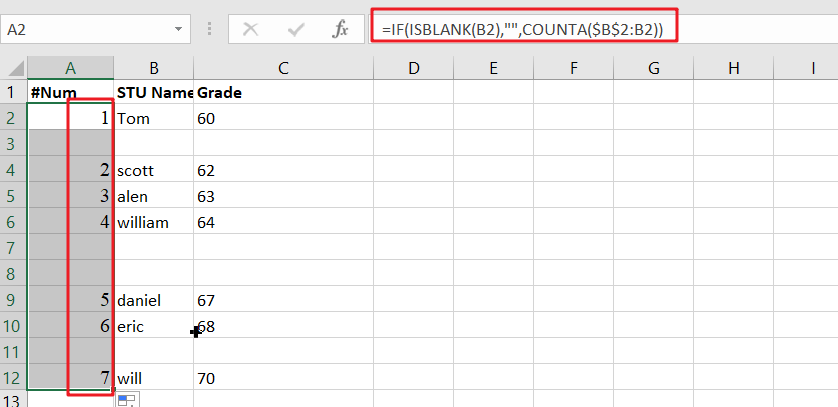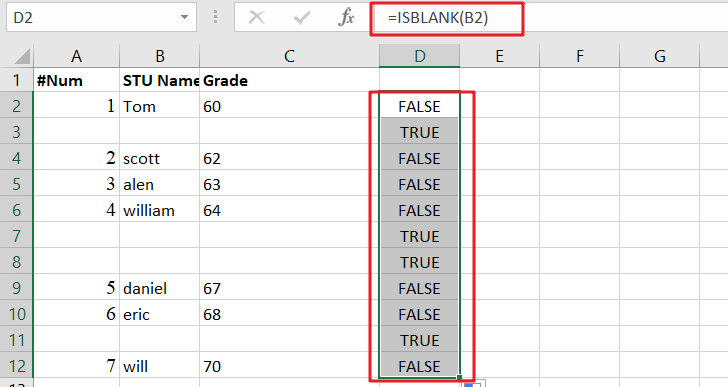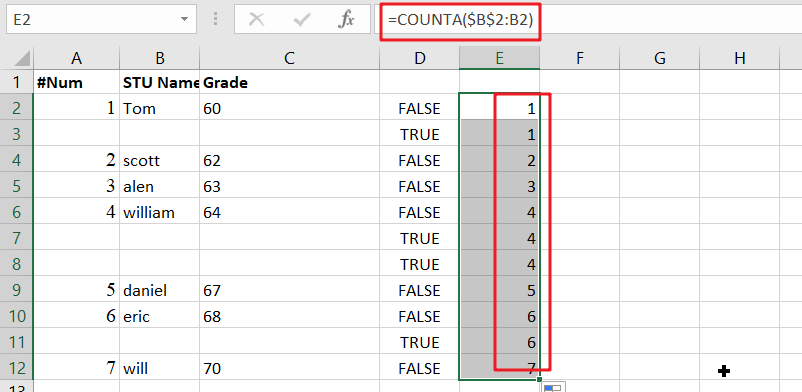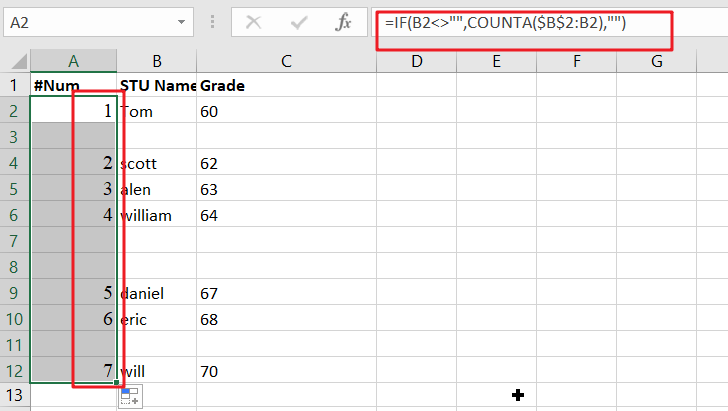# Add Row Numbers And Skip Blanks in Excel

Do you ever have to input a list of numbers into a spreadsheet, and some of the cells are blank? It can be difficult to keep track of what number is in which cell when you have to scroll up and down the sheet to look for the right spot. Well, today, I’m going to show you how to add row numbers and skip blanks so that your data entry process is a little bit smoother. Let’s get started!## Generic formula

`=IF(ISBLANK(B2),"",COUNTA(\$B \$2: B 2))`

## Summary

To add row numbers to a list of data, skipping blank cells, you can use the formula based on COUNTA and ISBLANK. Just using the following formula:

`=IF(ISBLANK(B2),"",COUNTA(\$B\$2:B2))`A simple way is to use IF statements with anondata formulas to show certain information when it comes up while keeping other things hidden from view unless desired by users who will open them up specifically looking for these details themselves!

## Explanation

The purpose is to add row numbers in column A only when there’s a value present elsewhere.

The IF function checks whether or not cell B2 has any values with ISBLANK.

`=ISBLANK(B2) // FALSE if not, TRUE if empty`When B2 is empty, ISBLANK returns TRUE, and the IF function produces a plain string like this: “”. If there’s anything in between them (i.e., not an expandable reference), we get COUNTA with whatever values are stored at each cell of that array; namely, nothing if it exists or NaN for non-numbers.

`=COUNTA(\$B\$2:B2) // expanding range`As the formula is copied down, each row’s count expands to include all non-blank cells in that range. COUNTA will count both numbers and text, making it easy for you to determine who was counting what during your data entry!

## Method2: Autonumber Rows If Adjacent Cell Not Blank

The easiest way to auto number your column is by using this formula: =IF(B2<>””,COUNTA(\$B\$2:B2),””) and then dragging down with the filehandle. You can also add row numbers manually if you want, but why not make things easier on yourself?Note: When you enter a formula to auto number cells, make sure that B2 is populated with the relevant value.

Entering new data or deleting existing values will automatically cause the sequence number in column A to be renumbered.

### Related Functions

• Excel ISBLANK function
The Excel ISBLANK function returns TRUE if the value is blank or null.The syntax of the ISBLANK function is as below:= ISBLANK (value)…
• Excel IF function
The Excel IF function perform a logical test to return one value if the condition is TRUE and return another value if the condition is FALSE. The IF function is a build-in function in Microsoft Excel and it is categorized as a Logical Function.The syntax of the IF function is as below:= IF (condition, [true_value], [false_value])….
• Excel COUNTA function
The Excel COUNTA function counts the number of cells that are not empty in a range. The syntax of the COUNTA function is as below:= COUNTA(value1, [value2],…)…